# Long Division by Monica Yuskaitis Long Division Long

• Slides: 19Long Division by Monica YuskaitisLong Division • Long division is as simple as memorizing the people in this family. Dad Mom Sister Rover Brother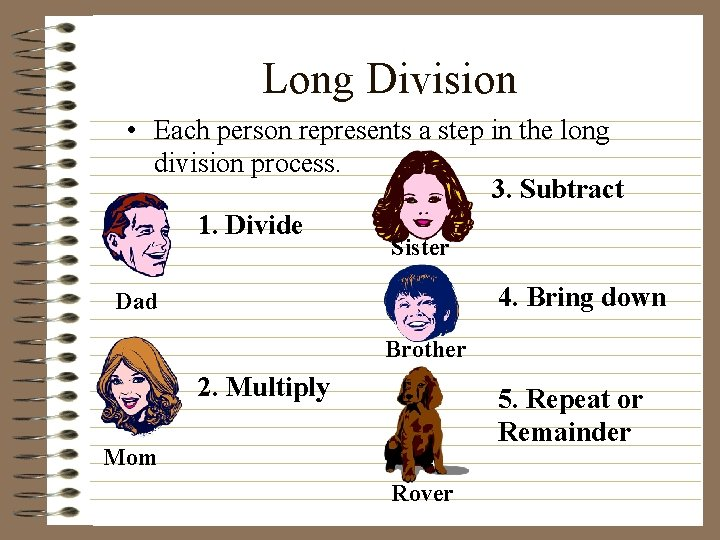Long Division • Each person represents a step in the long division process. 3. Subtract 1. Divide Sister 4. Bring down Dad Brother 2. Multiply 5. Repeat or Remainder Mom Rover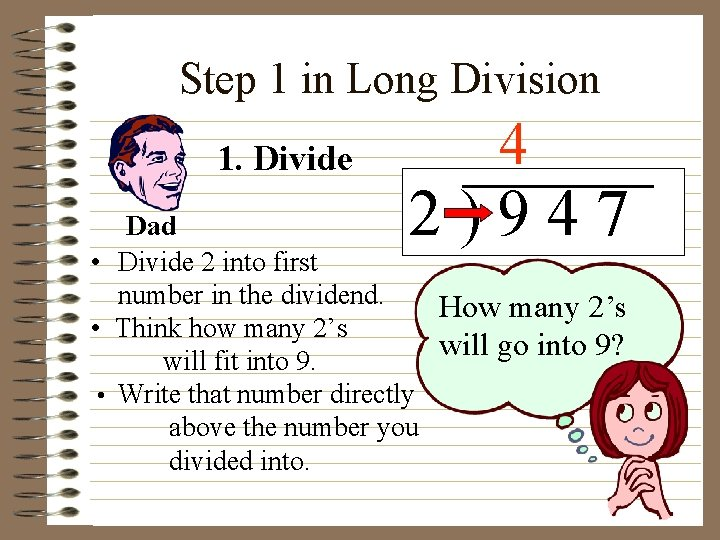Step 1 in Long Division 1. Divide 4 2)947 Dad • Divide 2 into first number in the dividend. How many 2’s • Think how many 2’s will go into 9? will fit into 9. • Write that number directly above the number you divided into.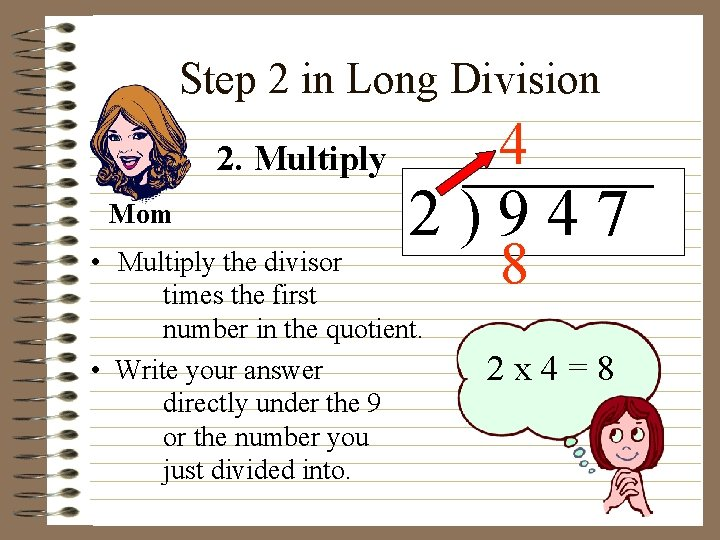Step 2 in Long Division 2. Multiply Mom 4 2)947 • Multiply the divisor times the first number in the quotient. • Write your answer directly under the 9 or the number you just divided into. 8 2 x 4=8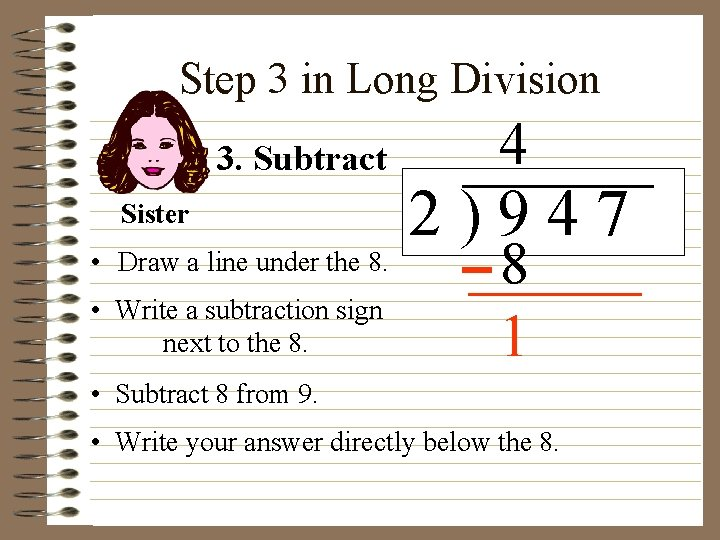Step 3 in Long Division 3. Subtract Sister • Draw a line under the 8. • Write a subtraction sign next to the 8. 4 2)947 8 1 • Subtract 8 from 9. • Write your answer directly below the 8.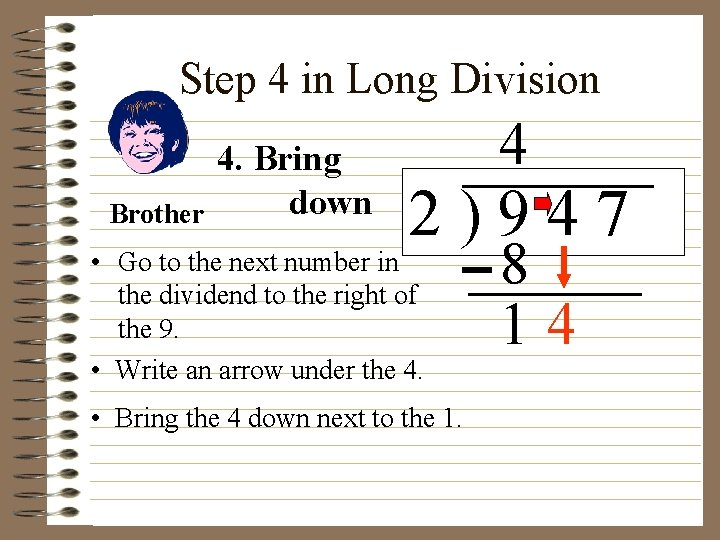Step 4 in Long Division Brother 4. Bring down 4 2)947 • Go to the next number in the dividend to the right of the 9. • Write an arrow under the 4. • Bring the 4 down next to the 1. 8 14Step 5 in Long Division 5. Repeat or Remainder 4 2)947 Rover • This is where you decide whether you repeat the 5 steps of division. • If your divisor can divide into your new number, 14, or if you have numbers in the dividend that have not been brought down, you repeat the 5 steps of division. 8 14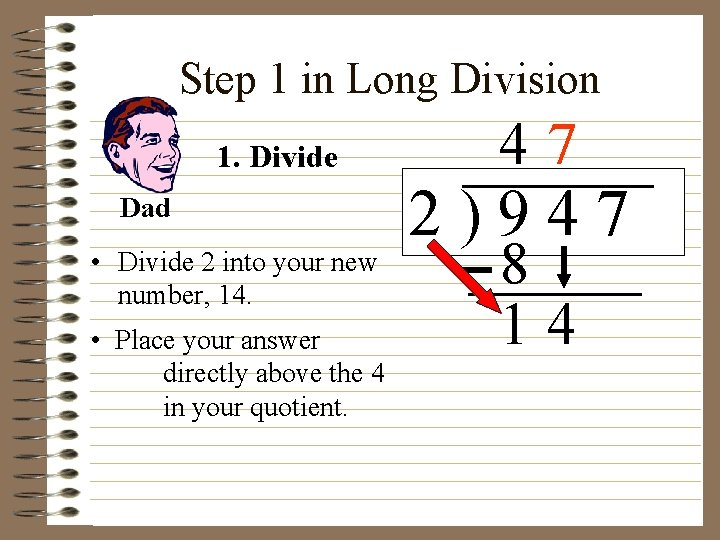Step 1 in Long Division 1. Divide Dad • Divide 2 into your new number, 14. • Place your answer directly above the 4 in your quotient. 47 2)947 8 14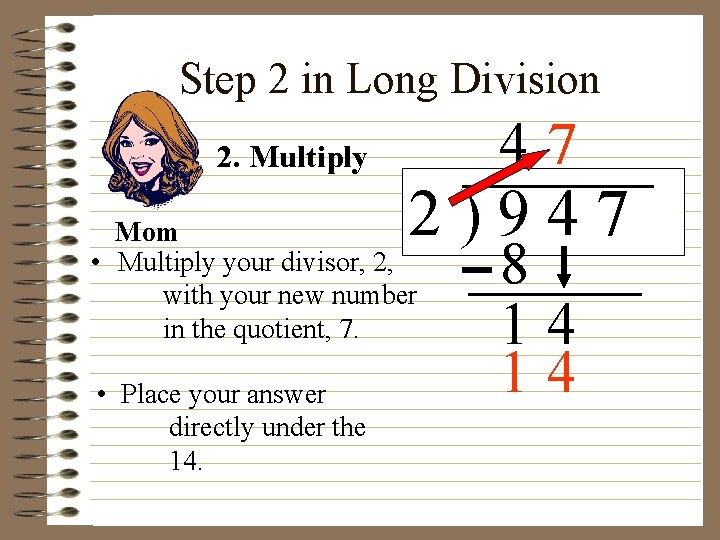Step 2 in Long Division 47 2. Multiply 2)947 Mom • Multiply your divisor, 2, with your new number in the quotient, 7. • Place your answer directly under the 14. 8 14 14Step 3 in Long Division 3. Subtract Sister • Draw a line under the bottom 14. • Draw a subtraction sign. • Subtract & place answer under the line. 47 2)947 8 14 14 0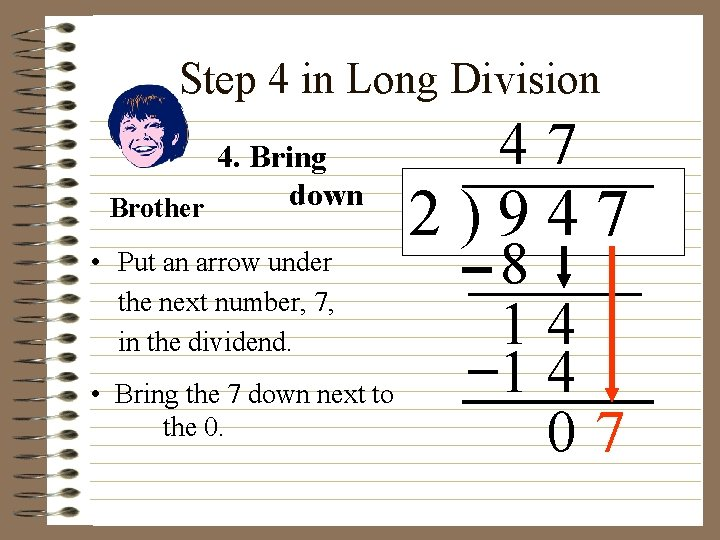Step 4 in Long Division 4. Bring down Brother • Put an arrow under the next number, 7, in the dividend. • Bring the 7 down next to the 0. 47 2)947 8 14 14 07Step 5 in Long Division Rover 5. Repeat or Remainder 47 2)947 • If the 2 will divide into your new number, 7, then repeat the steps of division. 8 14 14 07Step 1 in Long Division 47 3 1. Divide Dad 2)947 • Divide your divisor, 2, into your new number, 7. • Place your answer in the quotient next to the 7. 8 14 14 07Step 2 in Long Division 2. Multiply Mom 47 3 2)947 • Multiply your divisor, 2, by your new number in the quotient, 3. • Place your answer under the number you brought down, 7. 8 14 14 07 6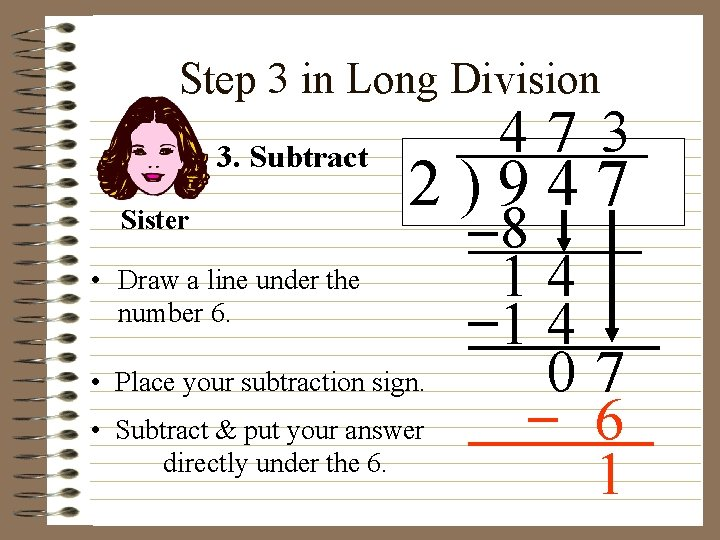Step 3 in Long Division 3. Subtract Sister 47 3 2)947 • Draw a line under the number 6. • Place your subtraction sign. • Subtract & put your answer directly under the 6. 8 14 14 07 6 1Step 4 in Long Division Brother 4. Bring down 2)947 • Look at your dividend to see if there any more numbers to bring down. • If not, move to step 5. 47 3 8 14 14 07 6 1Step 5 in Long Division 5. Repeat or Remainder Rover 47 3 2)947 • Since there are no more numbers to bring down & 2 will not divide into 1, you do not repeat the steps of division. • The number left over, 1, becomes the remainder. 8 14 14 07 6 1 R 1You did it! 47 3 2)947 You’re so smart! 8 14 14 07 6 1 R 1 You’re awesome! Cool Dude! Wolf!